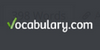### factorization[n] - (mathematics) the resolution of an integer or polynomial into factors such that when multiplied together they give the integer or polynomial
Found on http://www.webdictionary.co.uk/definition.php?query=factorization

### factorizationfactorisation noun (mathematics) the resolution of an integer or polynomial into factors such that when multiplied together they give the integer or polynomial
Found on https://www.encyclo.co.uk/local/20974

### FactorizationIn mathematics, factorization (also factorisation in British English) or factoring is the decomposition of an object (for example, a number, a polynomial, or a matrix) into a product of other objects, or factors, which when multiplied together give the original. For example, the number 15 factors into primes as 3 × 5, and the polynomial x2 − 4 ...
Found on http://en.wikipedia.org/wiki/Factorization

### factorization(mathematics) the resolution of an entity into factors such that when
Found on https://www.vocabulary.com/lists/310894
No exact match found